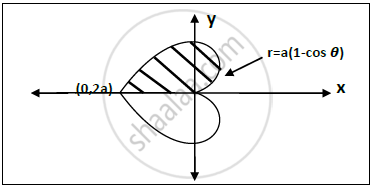Advertisement Remove all ads

# Find the Perimeter of the Curve R=A(1-cos 𝜽) - Applied Mathematics 2

Sum

Find the perimeter of the curve r=a(1-cos 𝜽)

Advertisement Remove all ads

#### Solution

Curve : r=a(1-cos 𝜽)Perimeter of given curve is ,

s=2xxint_0^pisqrt(r^2+((dr)/(d theta))^2d theta)

(dr)/(d theta)=a(sin theta)=>((dr)/(d theta))^2=a^2sin^2theta

r^2+((dr)/(d theta))^2=a^2[1-2cos theta+cos^2 theta]+a^2sin^2theta

sqrt(r^2+((dr)/(d theta))^2)=sqrt(2a)(1-cos theta)^(1/2)

=sqrt(2a)sqrt2sin(theta/2)

therefore s = 2int_0^pisqrt(2a)sqrt2sin(theta/2)d theta

=4aint_0^pisin(theta/2)d theta

=4a[-2cos(theta/2)]_0^pi

∴ S = 8a

Concept: Rectification of Plane Curves
Is there an error in this question or solution?
Advertisement Remove all ads

#### APPEARS IN

Advertisement Remove all ads
Advertisement Remove all ads
Share
Notifications

View all notifications

Forgot password?